#Engineering Mathematics Six Law of Indices pdf

An online surds rule printable

# Six rules of the Law of Indices:

To manipulate math expressions, we can consider using the Law of Indices. These laws only apply to expressions with the same base, for example, 34 and 32 can be manipulated using the Law of Indices, but we cannot use the Law of Indices to manipulate the expressions 45 and 97 as their base differs (their bases are 4 and 9, respectively).

 Rule 1: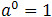Rule 2: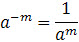Rule 3: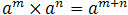Rule 4: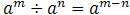Rule 5: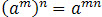Rule 6: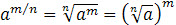## Example for 6 rules of the Law of Indices

Example for Rule 1:Simplify 20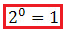Example for Rule 2:Simplify 2-2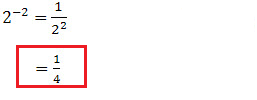Example for Rule 3:Simplify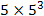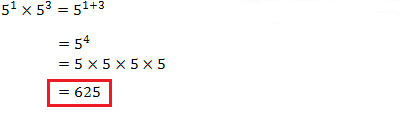Example for Rule 4:Simplify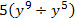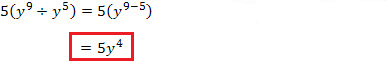Example for Rule 5:Simplify (y2)6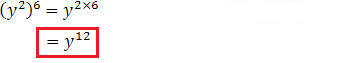Example for Rule 6:Simplify 1252/3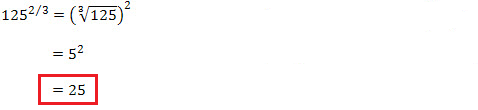##Age Calculators ►

Horse Age Calculator , Cow Age Calculator , Cat Age Calculator , Dog Age Calculator, Human Age Calculator 100 Days Old Calculator Date Difference Calculator